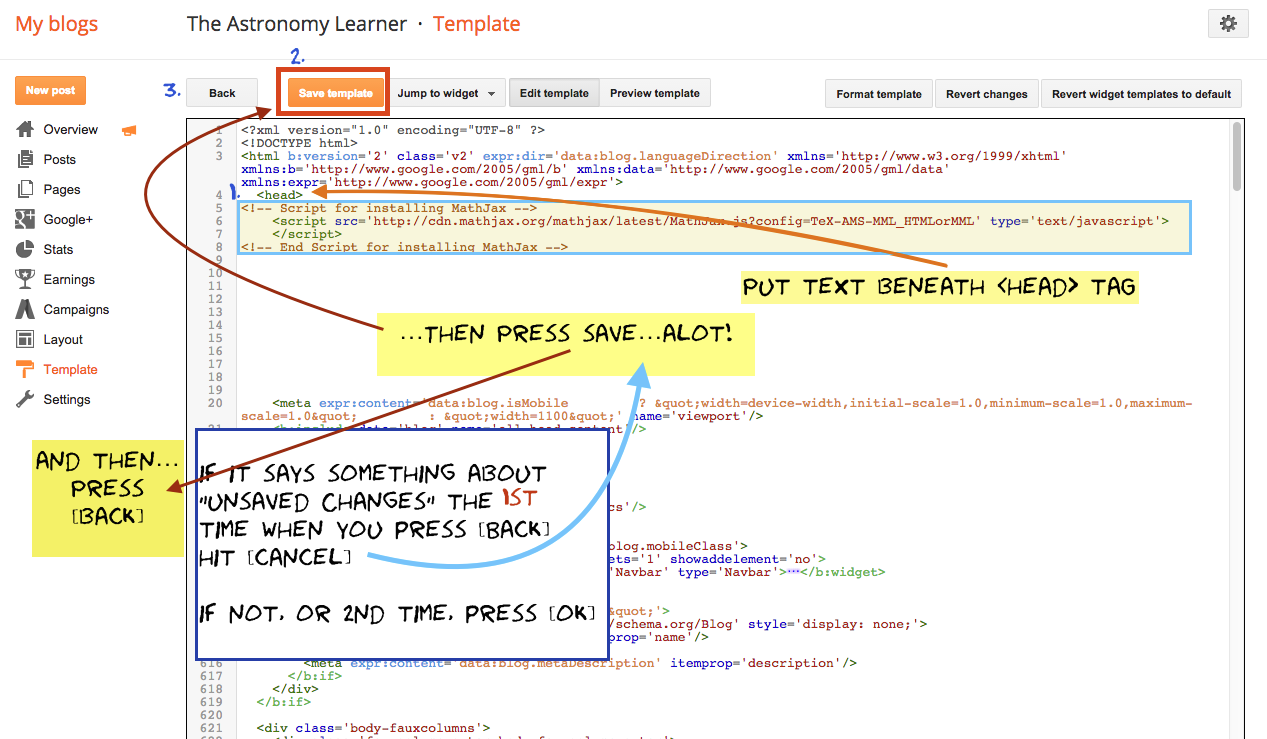MathJax on Blogger

Typesetting mathematics in HTML is time-consuming to say the least. The LaTeX language math-mode allows us to typeset math in a much simpler way. Our interface to this system is MathJax. Setting up MathJax on Blogger can be a daunting task. While there are many ways to implement MathJax on the internet. You will want to put the following code into the Blogger template you choose to use. Paste the code right below the head tag in the template. To do this go to the Template Editor and edit the HTML (Template >> Edit HTML)

The simplest method is to just use the paste the following code into the template:After pasting, make sure none of the text or symbols have changed. If it is not working, sometimes it is a good idea to paste it into a plain-text text editor (like EditPad).

The config=Tex-AMS-MML-HTMLorMML tells MathJax what packages and extensions to load by default. What we are really interested in here is the “AMS” portion. This provides access to the suite of symbols provided by the AMS LaTeX package. Symbols is Table 1 are not available within MathJax.

Inline math uses $$...$$ and display math is $...$. For example $$M_\odot$$ is $$M_\odot$$ and $a = \frac{G M}{r^2}$ is $a = \frac{G M}{r^2}$.

More information on configuring MathJax can be found on the MathJax website here and here